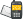It is currently 24 Nov 2020, 23:34### GMAT Club Daily Prep

#### Thank you for using the timer - this advanced tool can estimate your performance and suggest more practice questions. We have subscribed you to Daily Prep Questions via email.

Customized
for You

we will pick new questions that match your level based on your Timer History

Track

every week, we’ll send you an estimated GMAT score based on your performance

Practice
Pays

we will pick new questions that match your level based on your Timer History

#### Not interested in getting valuable practice questions and articles delivered to your email? No problem, unsubscribe here.# Equilater trinagle PQR is formed by joiningQuestion banks Downloads My Bookmarks Reviews Important topics
Author Message
TAGS:FounderJoined: 18 Apr 2015
Posts: 13891
GRE 1: Q160 V160Followers: 313

Kudos [?]: 3676  , given: 12912

Equilater trinagle PQR is formed by joining [#permalink]
1
KUDOS
Expert's post00:00

Question Stats:68% (00:44) correct31% (00:55) wrongbased on 147 sessions
Attachment:#GREpracticequestion Equilateral triangle PQR is formed by joining.jpg [ 17.91 KiB | Viewed 3831 times ]

Equilateral triangle PQR is formed by joining center P, Q, and R of the circles. Each pair of circles has exactly one point in common.

 Quantity A Quantity B The perimeter of triangle PQR The circumference of the circle with center Q

A) Quantity A is greater.
B) Quantity B is greater.
C) The two quantities are equal.
D) The relationship cannot be determined from the information given.
[Reveal] Spoiler: OA

Last edited by Carcass on 14 Jan 2019, 14:48, edited 3 times in total.
Edited the OAManagerJoined: 15 Feb 2018
Posts: 53
Followers: 1

Kudos [?]: 25  , given: 33

Re: Equilater trinagle PQR is formed by joining [#permalink]
1
KUDOS

A: Let r be the radius of each circle. Each side of the equilateral triangle is 2r, so the perimeter is 6r.

B: Circumference of Q = 2 * 3.14 * r > 6r.InternJoined: 05 Jun 2017
Posts: 8
Followers: 0

Kudos [?]: 8  , given: 0

Re: Equilater trinagle PQR is formed by joining [#permalink]
1
KUDOS
From given info
Rp + Rq = other two sides of the triangle
So, perimeter will be 3(Rp+Rq)
Now we have to compare
3Rp + 3Rq ? 2 Pi Rq

As we don't know the value of Rp so answer can not be determined.InternJoined: 14 Apr 2018
Posts: 7
Followers: 0

Kudos [?]: 5  , given: 5

Re: Equilater trinagle PQR is formed by joining [#permalink]
1
KUDOS
Should the answer be B for this question?
FounderJoined: 18 Apr 2015
Posts: 13891
GRE 1: Q160 V160Followers: 313

Kudos [?]: 3676 , given: 12912

Re: Equilater trinagle PQR is formed by joining [#permalink]
Expert's post
Sorry for the inconvenience. fixed.

regards
_________________
InternJoined: 02 Apr 2018
Posts: 9
Followers: 0

Kudos [?]: 1 , given: 3

Re: Equilater trinagle PQR is formed by joining [#permalink]
Yep the answer is b as the triangle is equilateral
InternJoined: 08 Apr 2018
Posts: 44
Followers: 0

Kudos [?]: 17 , given: 15

Re: Equilater trinagle PQR is formed by joining [#permalink]
Carcass wrote:
Sorry for the inconvenience. fixed.

regards

This is yet to be fixed as the answer placed is B,D. Which exactly is it?ManagerJoined: 02 May 2018
Posts: 58
Followers: 0

Kudos [?]: 32  , given: 22

Re: Equilater trinagle PQR is formed by joining [#permalink]
1
KUDOS
Emike56 wrote:
Carcass wrote:
Sorry for the inconvenience. fixed.

regards

This is yet to be fixed as the answer placed is B,D. Which exactly is it?

Here's another explanation why. Let's pretend that the radius (r) = 5.
Since the triangle is an equilateral triangle, that means each side of the triangle is 10, or 2 * radius of each circle.

Quantity A = Perimeter of Triangle PQR = 30.
Quantity B = Circumference of Circle with Center Q = 2 * pi * 5. = 10 * 3.14 = 31.4.

Quantity B is greater.

DirectorJoined: 09 Nov 2018
Posts: 505
Followers: 0

Kudos [?]: 56 , given: 1

Re: Equilater trinagle PQR is formed by joining [#permalink]
Ans B
InternJoined: 30 Jul 2018
Posts: 3
Followers: 0

Kudos [?]: 2 , given: 6

Re: Equilater trinagle PQR is formed by joining [#permalink]
Agree, the answer is B if the circles are identical but there is nowhere mentioned in the question that the circles have the same radius.

Thanks
FounderJoined: 18 Apr 2015
Posts: 13891
GRE 1: Q160 V160Followers: 313

Kudos [?]: 3676 , given: 12912

Re: Equilater trinagle PQR is formed by joining [#permalink]
Expert's post
The assumption is wrong: the stem says that PQR is an equilateral triangle, which means all sides are equal in length.

So, if one side is 10 (for instance) the other two are 10 as well.

If one side is 10, half of it is 5, which is the radius of all 3 circles. Is the radius is equal, the 3 areas are equal as well.

Hope now is clear.

Regards
_________________
InternJoined: 19 Jul 2020
Posts: 2
Followers: 0

Kudos [?]: 0 , given: 2

Re: Equilater trinagle PQR is formed by joining [#permalink]
How do you know all Circles are equal?

Posted from my mobile deviceFounderJoined: 18 Apr 2015
Posts: 13891
GRE 1: Q160 V160Followers: 313

Kudos [?]: 3676 , given: 12912

Re: Equilater trinagle PQR is formed by joining [#permalink]
Expert's post
Because the equilateral triangle has 3 side equal in lenght

The same side, added up. is the two radii of a circle.

Therefore the circles must be equal
_________________Re: Equilater trinagle PQR is formed by joining   [#permalink] 31 Aug 2020, 09:46
Display posts from previous: Sort by

# Equilater trinagle PQR is formed by joiningQuestion banks Downloads My Bookmarks Reviews Important topicsPowered by phpBB © phpBB Group Kindly note that the GRE® test is a registered trademark of the Educational Testing Service®, and this site has neither been reviewed nor endorsed by ETS®.